Courses

# Test: Control System- 2

## 25 Questions MCQ Test Basic Electronics Engineering for SSC JE (Technical) | Test: Control System- 2

Description
This mock test of Test: Control System- 2 for Electrical Engineering (EE) helps you for every Electrical Engineering (EE) entrance exam. This contains 25 Multiple Choice Questions for Electrical Engineering (EE) Test: Control System- 2 (mcq) to study with solutions a complete question bank. The solved questions answers in this Test: Control System- 2 quiz give you a good mix of easy questions and tough questions. Electrical Engineering (EE) students definitely take this Test: Control System- 2 exercise for a better result in the exam. You can find other Test: Control System- 2 extra questions, long questions & short questions for Electrical Engineering (EE) on EduRev as well by searching above.
QUESTION: 1

Solution:
QUESTION: 2

Solution:
QUESTION: 3

###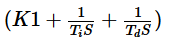Solution:
QUESTION: 4

The industrial controller having the best steady -state accuracy is

Solution:
QUESTION: 5

consider the following statements :
1. The effect of feedback is to reduce the system error
2. Feedback increases the gain of the system in one frequency range but decreases in another.
3. Feedback can cause a system that is originally stable to become unstable

Which of these statements are correct ?

Solution:
QUESTION: 6

The intersection of asymptotes of root -loci of a system with open loop transfer function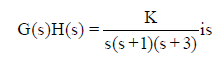Solution:
QUESTION: 7

The open-loop transfer function of unity feedback control system is
The system is stable is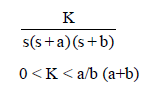Solution:
QUESTION: 8

Which one of the following characteristicequations of result in the stable operation of thefeedback system ?

Solution:
QUESTION: 9

Consider the following statements:

Routh-Hurwitz critierion gives
1. aboslute stability
2. the number of roots lying on the right half of the s-plane
3. the gainmargin and phase margin

Which of these statements are correct ?

Solution:
QUESTION: 10

A second order system has the damping ratio xand undamped natural frequency of oscillation wn.The setting time at 2% tolerance band of thesystem is

Solution:
QUESTION: 11

Consider the following statements:
A proportional plus derivative controller
1. has high sensitivity
2. increases the stability of the system

Which of these statements are correct ?

Solution:
QUESTION: 12

The open loop transfer function of a system is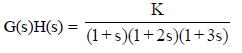The phase crossover frequency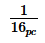is

Solution:
QUESTION: 13

Open loop transfer function of a system havingone zero with a positive real value is called

Solution:
QUESTION: 14

The given characteristic polynomial
s4 + s3 + 2s2 + 2s + 3 =0 has

Solution:
QUESTION: 15

Which one of the following is the steady-state error for a step input applied to a unity feedbook system with the open loop transfer Function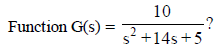Solution:
QUESTION: 16

The open -loop transfer function of a unity feedback control system is given as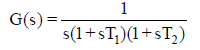The phase crossover frequency and the gain margin are, respectively (Correct the options)

Solution:
QUESTION: 17

The transfer function of a phase lead network can be written as

Solution:
QUESTION: 18

A control system has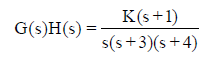Root locus of the system can lie on the real axis

Solution:
QUESTION: 19

Consider the following open loop frequency response of a unit feedback system: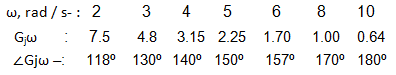The gain and phase margin of the system arerespectively

Solution:
QUESTION: 20

The transfer function of a phase lead network, as shown in the figure below is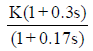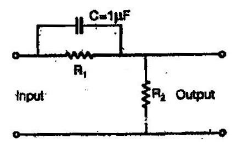The values, of R1 and R2 are respectively

Solution:
QUESTION: 21

The forward path transfer function of a unity feedback system is given by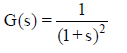What is the phase margin for this system ?

Solution:
QUESTION: 22

Which one of the following statement is correct?

The effects of phase lead compensator on gain crossover frequency (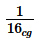) and the bandwidth(BW) are

Solution:
QUESTION: 23

What is the unit step response of a unity feedback control system having forward path transfer function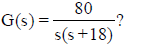Solution:
QUESTION: 24

An open loop system has a transferfunction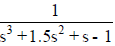. it is converted into aclosed loop system by providing a negative feedback having transfer function 20 (s+ 1).Which one of the following is correct ?The open loop and closed loop systems are,respectively

Solution:
QUESTION: 25

With regard to the filtering property, the leadcompensator and the lag compensator are,respectively.

Solution: